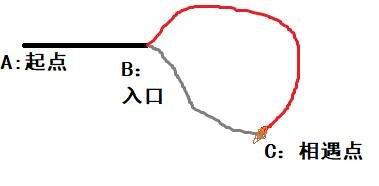## 题目1：机器人的运动范围

1.设置标志位。bool flags=new bool[rowscols]。

2.将移动一步单独设置为一个子函数，在这个函数里面，原始的判断条件只是：

if(i>=0 && i< rows && j >=0 && j<cols && flags[i*cols+j]==false)

if(i>=0 && i< rows && j >=0 && j<cols && (gssum(i) + gssum(j) <= threshold) && flags[i*cols+j]==false)

int movingCount(int threshold, int rows, int cols) { bool *flags=new bool[rows*cols];//所有格子的标志位默认设置为false for(int i=0;i<rows*cols;i++) flags[i]=false; int count = movingstep(threshold, rows, cols, 0, 0, flags);;//记录可访问格子数量 return count; } int movingstep(int threshold, int rows, int cols, int i, int j, bool *flags) { int count = 0; if(i>=0 && i< rows && j >=0 && j<cols && (gssum(i) + gssum(j) <= threshold) && flags[i*cols+j]==false) { flags[i*cols+j]=true; count = 1 + movingstep(threshold, rows, cols, i+1, j, flags)+movingstep(threshold, rows, cols, i-1, j, flags)+movingstep(threshold, rows, cols, i, j+1, flags)+movingstep(threshold, rows, cols, i, j-1, flags); } return count; } int gssum(int i) { int sum = 0; while(i) { sum += i % 10;; i /= 10; } return sum; }

## 题目2：把字符串转换成整数

1.不考虑最前面的空格；

2.若除去空格后的第一位为“+”或“-”，则分别将标志位做不同的设置，若为数字，直接将标志位设置为同“+”，若为其它则直接返回0；

3.后续几位直接判断是否为0~9之间的数字，由于是字符串格式，所以为ascii码，这样，我们在转换成int格式的时候，直接用该字符减去’0’即可。

 int StrToInt(string str) { int len=str.length(); if(len==0) return 0; int i = 0; int res = 0; int fla = 1; //滤掉前面的空格 while(str[i]==' '){ i++; } //进行符号判断 if(str[i]=='+'){ i++; }else if(str[i]=='-'){ i++; fla=-1; } while(str[i]!='\0'){ //对字符进行是否为数字的判断 if(str[i]>='0'&&str[i]<='9'){ res=res*10+fla*(str[i]-'0'); i++; }else{ res=0; break; } } return res; }

## 题目3：表示数值的字符串

1.在数值之前可能有一个“+”或“-”，接下来是0到9的数位表示数值的整数部分，如果数值是一个小数，那么小数点后面可能会有若干个0到9的数字。

2表示数值的小数部分，如果用科学计数法表示，接下来是一个‘e’或者‘E’，以及紧跟着一个整数（可以有正负号）表示指数。

bool isNumeric(char* string) { if(string == NULL) return false; if(*string == '+' || *string =='-') string++; if(*string =='\0') return false; int dot = 0;//小数点的个数 int num = 0;//数字的个数 int ex = 0;//e指数的个数 while(*string !='\0') { if((*string >= '0') && (*string <='9')) { num++; string++; } //小数点开始 else if(*string =='.') { if(dot> 0 || ex > 0) return false; dot++; string++; } //指数e else if((*string =='e') ||(*string == 'E')) { if(num ==0 || ex > 0) return false; string++; ex++; if(*string == '+' || *string =='-') string ++; if(*string=='\0') return false; } else return false; } return true; }

## 题目4：链表中环的入口节点ListNode* EntryNodeOfLoop(ListNode* pHead) { if((pHead==NULL)||(pHead->next==NULL)||(pHead->next->next==NULL)) return NULL; ListNode* fast = pHead->next->next; ListNode* slow = pHead->next; //先判断有没有环 while(fast!=slow) { if((fast->next !=NULL) &&(fast->next->next!=NULL)){ fast=fast->next->next; slow=slow->next; } else{ return NULL; } } //如果能执行到这一步，说明有环，且此时fast==slow。 //然后把fast调整到头部，此时fast和slow第一次相遇的位置就是入口地址 fast=pHead; while(fast!=slow) { fast=fast->next; slow=slow->next; } return slow; }

## 题目5：把二叉树打印成多行

 vector<vector<int> > Print(TreeNode* pRoot) { vector<vector<int> > vect;//最后输出的结果 if(pRoot==NULL) return vect;//若pRoot为空，直接返回空 //接下来总体上就是一个层序遍历的变体，所以可以使用层序遍历进行修改完成此题目 //层序遍历可以利用队列来实现。 //首先新建一个队列，然后将链表的头push进去，然后弹出，获得头的值，然后再分别push进去其左右两个结点。然后弹出头上的 //然后判断其左右结点是否有，有就push进去。这样层序遍历其实就是用队列来输入输出。 queue<TreeNode*> tmp; tmp.push(pRoot); while(!tmp.empty()) { int l=0; int h=tmp.size(); vector<int> t; while(l++<h)//按行输出 { TreeNode* k = tmp.front(); tmp.pop(); t.push_back(k->val); if(k->left) tmp.push(k->left); if(k->right) tmp.push(k->right); } vect.push_back(t); } return vect; }

## 题目6：按之字形顺序打印二叉树

vector<vector<int> > Print(TreeNode* pRoot) { vector<vector<int> > res;//结果存放 stack<TreeNode*> stackl,stackr;//从右往左和从左往右输出结果的堆栈 if(pRoot!=NULL) { stackl.push(pRoot);//跟层序遍历类似，先把根节点push进去 } struct TreeNode* node;//中介的结点，类似于层序遍历 while(!stackl.empty() || !stackr.empty()) { vector<int> tmp; if(!stackl.empty()){ while(!stackl.empty()) { node=stackl.top(); stackl.pop(); tmp.push_back(node->val); if(node->left!=NULL) stackr.push(node->left); if(node->right!=NULL) stackr.push(node->right); } res.push_back(tmp); } else if(!stackr.empty()){ while(!stackr.empty()){ node=stackr.top(); stackr.pop(); tmp.push_back(node->val); if(node->right!=NULL) stackl.push(node->right); if(node->left!=NULL) stackl.push(node->left); } res.push_back(tmp); } } return res; }

## 题目7：二叉搜索树的第k个节点

class Solution {private: int count;public: void inorder(TreeNode* root, TreeNode* &ans) { if(root) { inorder(root->left,ans); count--;//记录是第几个值 if(!count) ans=root; inorder(root->right,ans); } } TreeNode* KthNode(TreeNode* pRoot, int k) { if(pRoot==NULL || k<1) return NULL; TreeNode* ans = NULL; count = k; inorder(pRoot, ans); return ans; }};

## 题目8：翻转单词顺序

void ReversedWord(string &str, int st, int en) { while(st < en) { swap(str[st++],str[en--]); } } string ReverseSentence(string str) { int len = str.size(); int st = 0; int en = 0; ReversedWord(str,0,len-1);//先整体翻转 int i=0; while(i < len) { while(i < len && str[i] == ' ') //空格跳过 i++; en = st = i; //记录单词的第一个字符的位置 while(i < len && str[i] != ' ') //不是空格 找单词最后一个字符的位置 { i++; en++; } ReversedWord(str, st, en - 1); //局部翻转 } return str; }

## 题目9：扑克牌顺子

LL今天心情特别好,因为他去买了一副扑克牌,发现里面居然有2个大王,2个小王(一副牌原本是54张^_^)…他随机从中抽出了5张牌,想测测自己的手气,看看能不能抽到顺子,如果抽到的话,他决定去买体育彩票,嘿嘿！！“红心A,黑桃3,小王,大王,方片5”,“Oh My God!”不是顺子…..LL不高兴了,他想了想,决定大\小 王可以看成任何数字,并且A看作1,J为11,Q为12,K为13。上面的5张牌就可以变成“1,2,3,4,5”(大小王分别看作2和4),“So Lucky!”。LL决定去买体育彩票啦。 现在,要求你使用这幅牌模拟上面的过程,然后告诉我们LL的运气如何， 如果牌能组成顺子就输出true，否则就输出false。为了方便起见,你可以认为大小王是0。

1.牌面不重复；

2.牌面是连续的。

1.设置flag。只要不重复，很多都会用到flag，由于扑克牌里面有14种牌，所以flag数组长度为14，默认为0，有重复就加1。

2.寻找最大最小值，计算它们的差值，判断结果。由于牌面不重复，又由于只有五张，所以max-min<5的时候（其实就是为4的时候），肯定是连续的，若大了，肯定不连续。

 bool IsContinuous( vector<int> numbers ) { if(numbers.empty()) return false; int count={0}; int max = -1; int min = 14; for(int i=0;i<numbers.size();i++) { count[numbers[i]]++; if(numbers[i]==0) continue; if(count[numbers[i]]>1) return false; if(numbers[i] > max) max = numbers[i]; if(numbers[i] < min) min = numbers[i]; } if(max - min <5) return true; return false; }

## 题目10：矩阵中的路径

private: bool isPath(char *matrix, vector<char> flags, char* str, int i, int j, int rows, int cols) { if(i<0|| i>=rows || j<0 || j>=cols) return false; if(matrix[i*cols+j] == *str && flags[i*cols+j]==0) { flags[i*cols+j]=1; if(*(str+1)==0) return true; bool cond = isPath(matrix,flags,(str+1),i,j-1,rows,cols) || isPath(matrix,flags,(str+1),i-1,j,rows,cols)|| isPath(matrix,flags,(str+1),i,j+1,rows,cols)|| isPath(matrix,flags,(str+1),i+1,j,rows,cols); if(cond==false) flags[i*cols+j]=0; return cond; } else return false; }public: bool hasPath(char* matrix, int rows, int cols, char* str) { vector<char> flags(rows*cols,0); bool con = false; for(int i=0;i<rows;i++) for(int j =0;j<cols;j++) { con = (con|| isPath(matrix,flags,str,i,j,rows,cols) ); } return con; }